Скачать презентацию CHAPTER 7 Bonds and Their Valuation n n

d8aeda43603bf86fd3bf9bcdaed375b1.ppt

• Количество слайдов: 43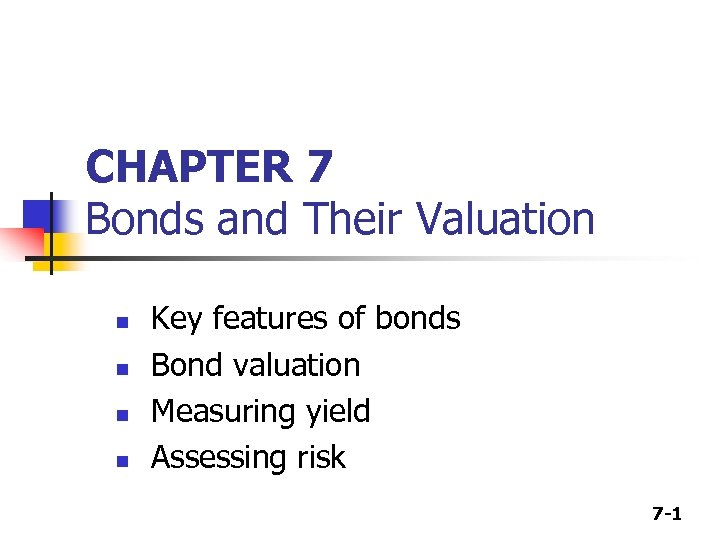CHAPTER 7 Bonds and Their Valuation n n Key features of bonds Bond valuation Measuring yield Assessing risk 7 -1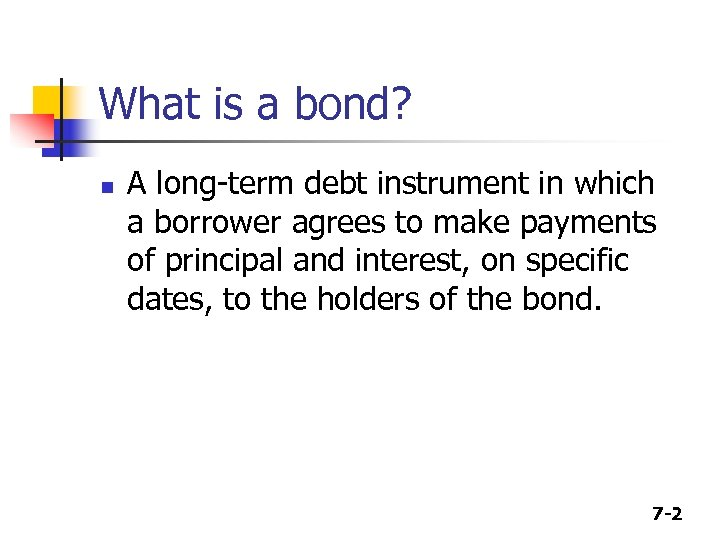What is a bond? n A long-term debt instrument in which a borrower agrees to make payments of principal and interest, on specific dates, to the holders of the bond. 7 -2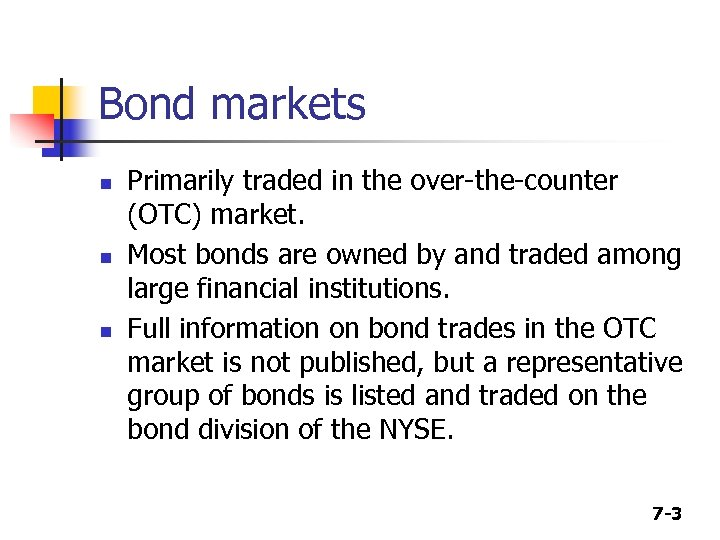Bond markets n n n Primarily traded in the over-the-counter (OTC) market. Most bonds are owned by and traded among large financial institutions. Full information on bond trades in the OTC market is not published, but a representative group of bonds is listed and traded on the bond division of the NYSE. 7 -3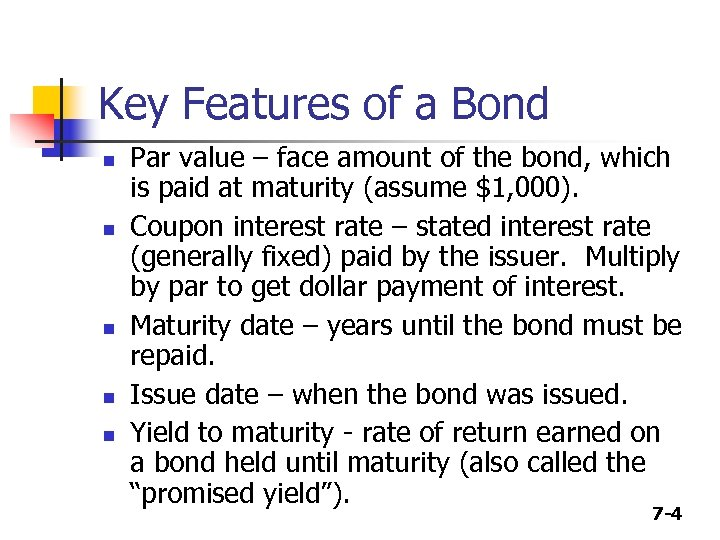Key Features of a Bond n n n Par value – face amount of the bond, which is paid at maturity (assume \$1, 000). Coupon interest rate – stated interest rate (generally fixed) paid by the issuer. Multiply by par to get dollar payment of interest. Maturity date – years until the bond must be repaid. Issue date – when the bond was issued. Yield to maturity - rate of return earned on a bond held until maturity (also called the “promised yield”). 7 -4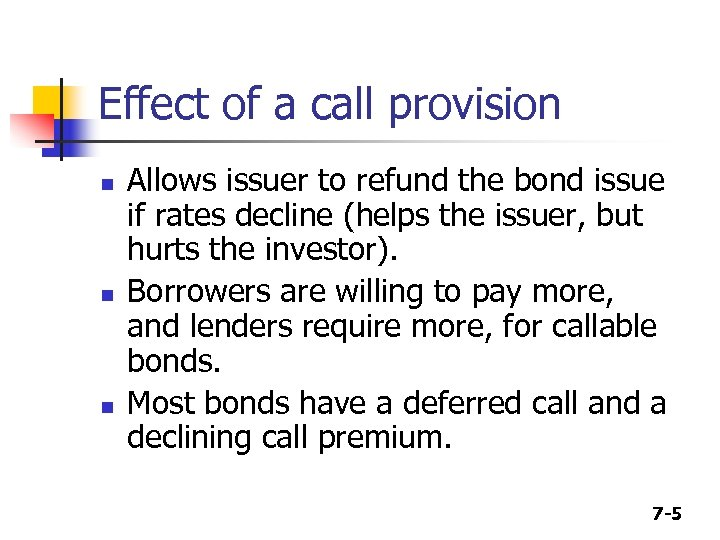Effect of a call provision n Allows issuer to refund the bond issue if rates decline (helps the issuer, but hurts the investor). Borrowers are willing to pay more, and lenders require more, for callable bonds. Most bonds have a deferred call and a declining call premium. 7 -5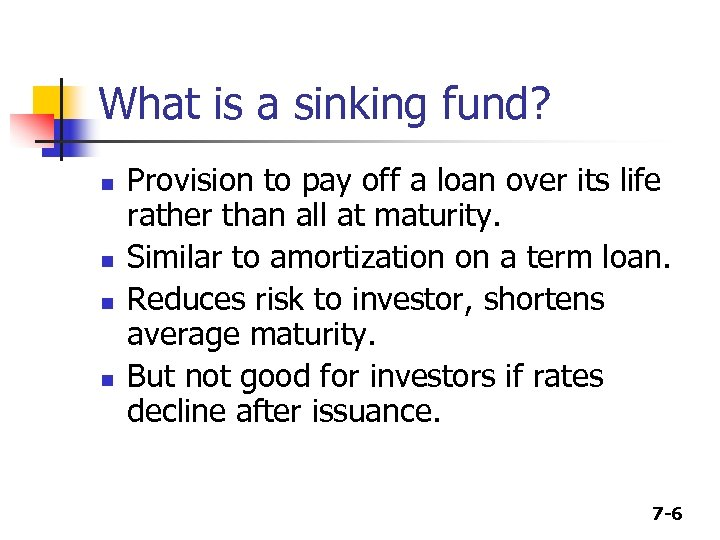What is a sinking fund? n n Provision to pay off a loan over its life rather than all at maturity. Similar to amortization on a term loan. Reduces risk to investor, shortens average maturity. But not good for investors if rates decline after issuance. 7 -6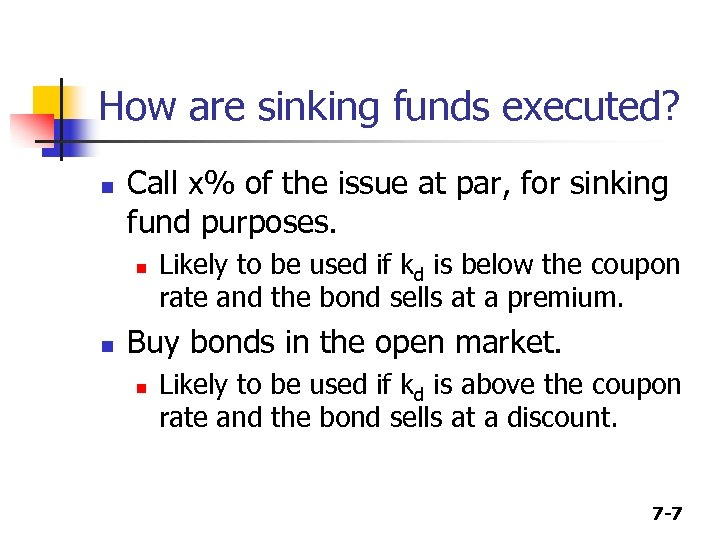How are sinking funds executed? n Call x% of the issue at par, for sinking fund purposes. n n Likely to be used if kd is below the coupon rate and the bond sells at a premium. Buy bonds in the open market. n Likely to be used if kd is above the coupon rate and the bond sells at a discount. 7 -7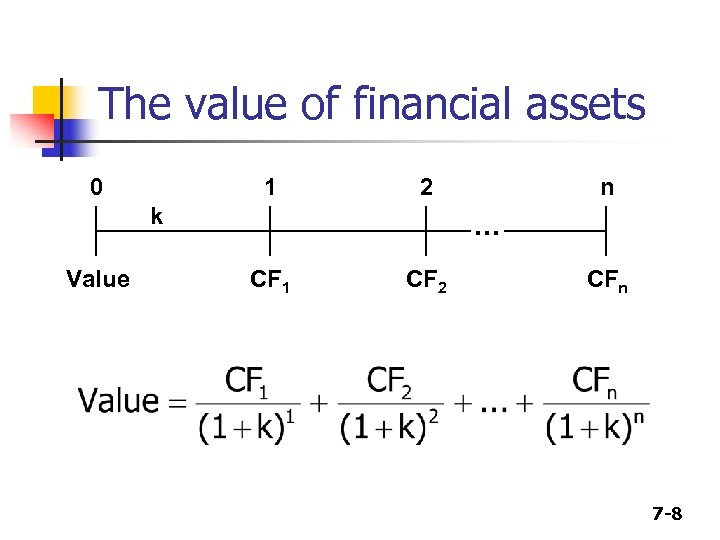The value of financial assets 0 1 2 k Value n . . . CF 1 CF 2 CFn 7 -8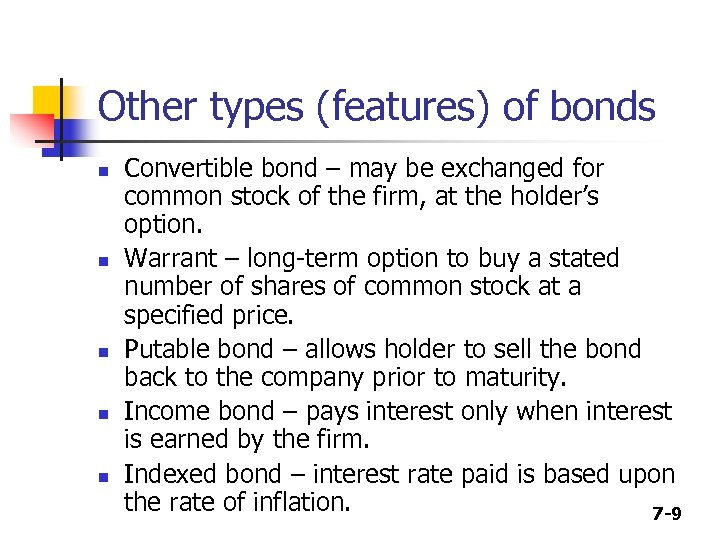Other types (features) of bonds n n n Convertible bond – may be exchanged for common stock of the firm, at the holder’s option. Warrant – long-term option to buy a stated number of shares of common stock at a specified price. Putable bond – allows holder to sell the bond back to the company prior to maturity. Income bond – pays interest only when interest is earned by the firm. Indexed bond – interest rate paid is based upon the rate of inflation. 7 -9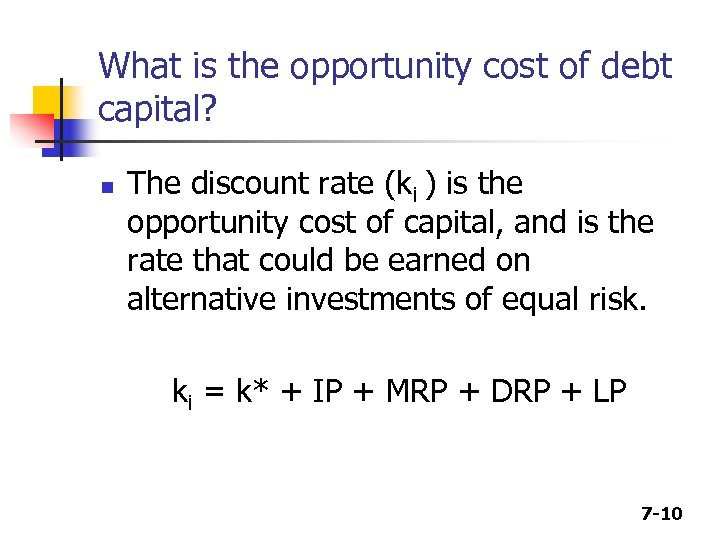What is the opportunity cost of debt capital? n The discount rate (ki ) is the opportunity cost of capital, and is the rate that could be earned on alternative investments of equal risk. ki = k* + IP + MRP + DRP + LP 7 -10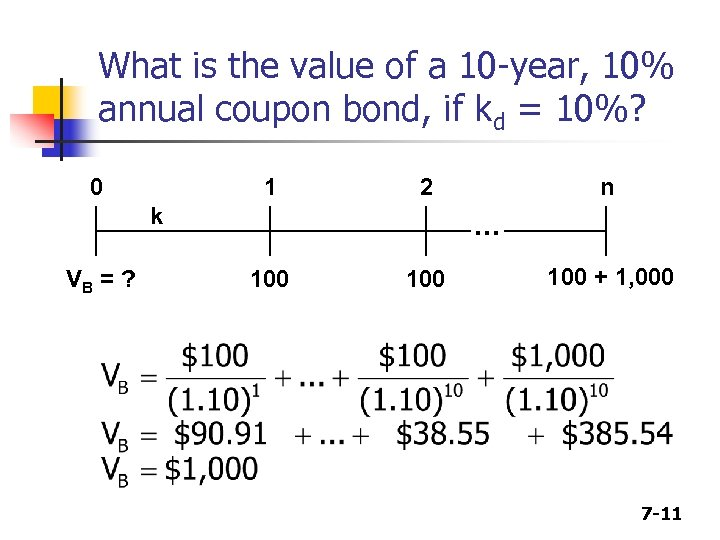What is the value of a 10 -year, 10% annual coupon bond, if kd = 10%? 0 1 2 k VB = ? n . . . 100 100 + 1, 000 7 -11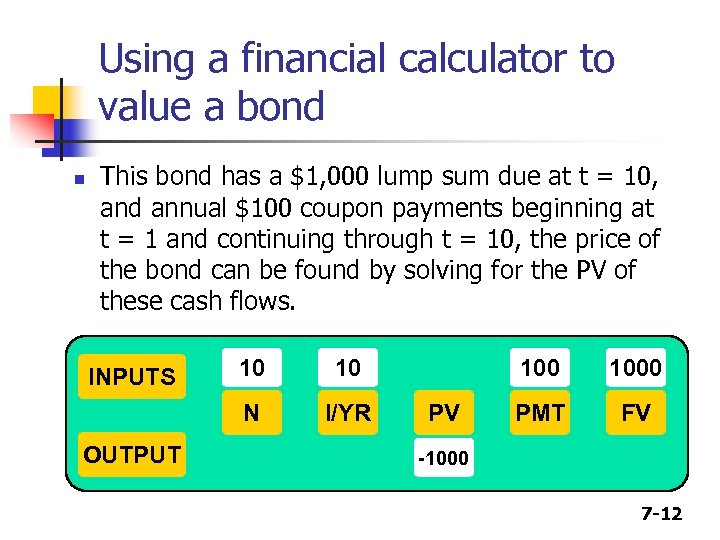Using a financial calculator to value a bond n This bond has a \$1, 000 lump sum due at t = 10, and annual \$100 coupon payments beginning at t = 1 and continuing through t = 10, the price of the bond can be found by solving for the PV of these cash flows. OUTPUT 10 10 N INPUTS I/YR 100 PV 1000 PMT FV -1000 7 -12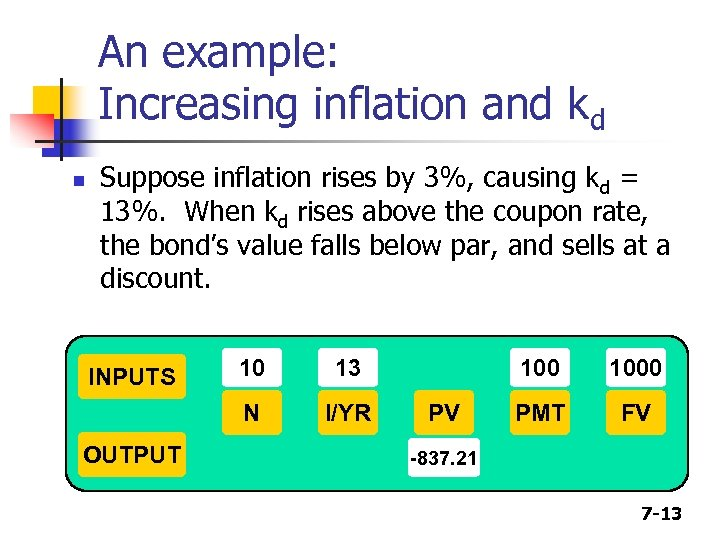An example: Increasing inflation and kd n Suppose inflation rises by 3%, causing kd = 13%. When kd rises above the coupon rate, the bond’s value falls below par, and sells at a discount. OUTPUT 10 13 N INPUTS I/YR 100 PV 1000 PMT FV -837. 21 7 -13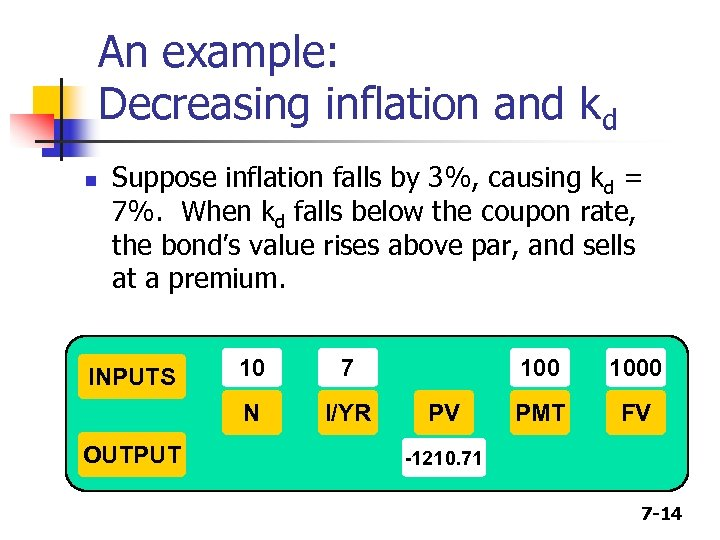An example: Decreasing inflation and kd n Suppose inflation falls by 3%, causing kd = 7%. When kd falls below the coupon rate, the bond’s value rises above par, and sells at a premium. OUTPUT 10 7 N INPUTS I/YR 100 PV 1000 PMT FV -1210. 71 7 -14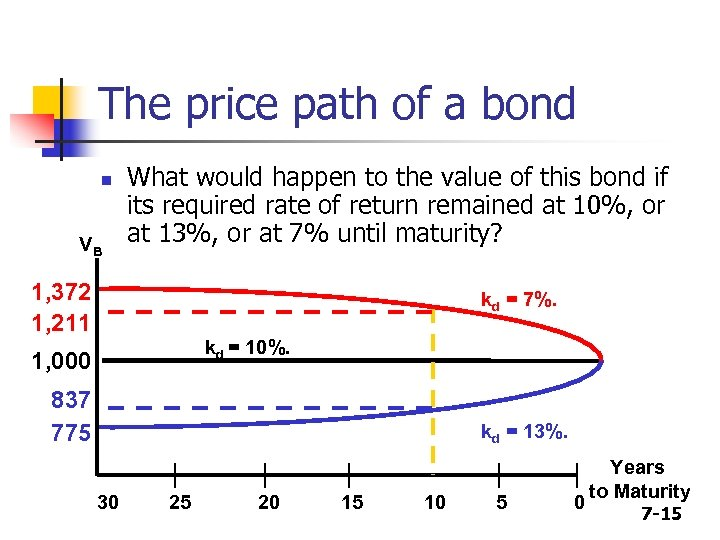The price path of a bond n VB What would happen to the value of this bond if its required rate of return remained at 10%, or at 13%, or at 7% until maturity? 1, 372 1, 211 kd = 7%. kd = 10%. 1, 000 837 775 kd = 13%. 30 25 20 15 10 5 0 Years to Maturity 7 -15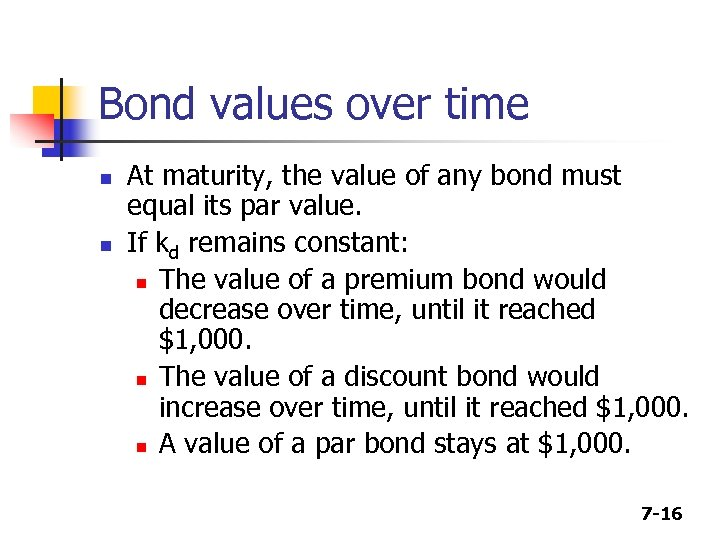Bond values over time n n At maturity, the value of any bond must equal its par value. If kd remains constant: n The value of a premium bond would decrease over time, until it reached \$1, 000. n The value of a discount bond would increase over time, until it reached \$1, 000. n A value of a par bond stays at \$1, 000. 7 -16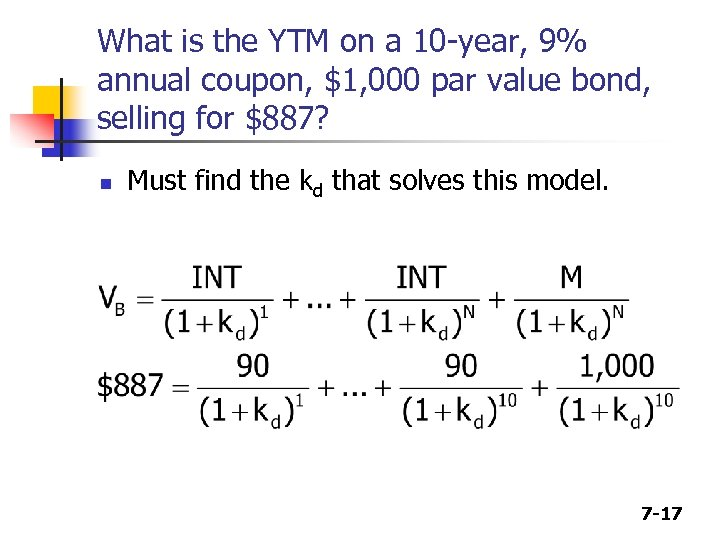What is the YTM on a 10 -year, 9% annual coupon, \$1, 000 par value bond, selling for \$887? n Must find the kd that solves this model. 7 -17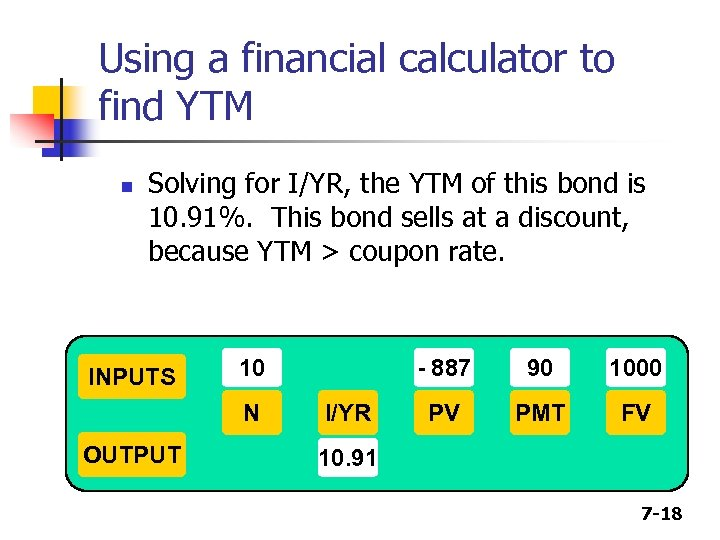Using a financial calculator to find YTM n Solving for I/YR, the YTM of this bond is 10. 91%. This bond sells at a discount, because YTM > coupon rate. INPUTS 10 N OUTPUT - 887 I/YR 90 1000 PV PMT FV 10. 91 7 -18Find YTM, if the bond price was \$1, 134. 20. n Solving for I/YR, the YTM of this bond is 7. 08%. This bond sells at a premium, because YTM < coupon rate. INPUTS 10 N OUTPUT -1134. 2 I/YR 90 1000 PV PMT FV 7. 08 7 -19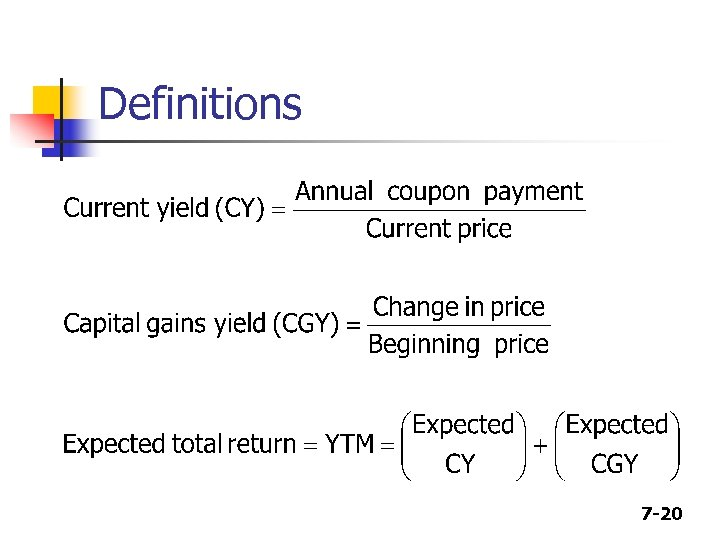Definitions 7 -20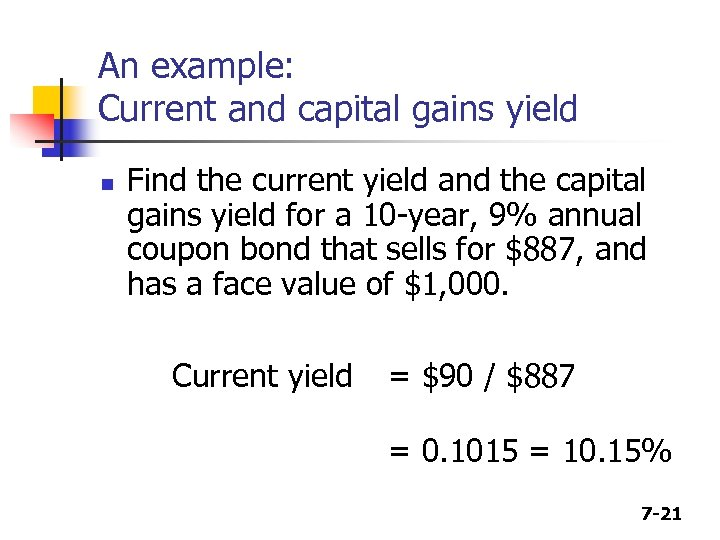An example: Current and capital gains yield n Find the current yield and the capital gains yield for a 10 -year, 9% annual coupon bond that sells for \$887, and has a face value of \$1, 000. Current yield = \$90 / \$887 = 0. 1015 = 10. 15% 7 -21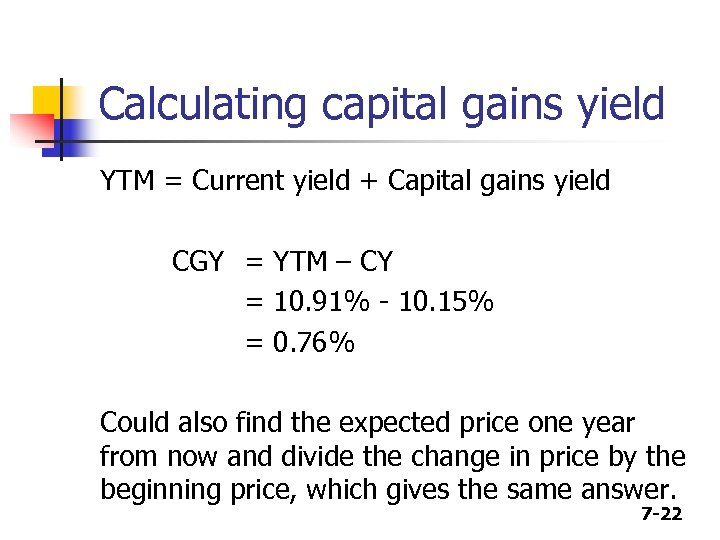Calculating capital gains yield YTM = Current yield + Capital gains yield CGY = YTM – CY = 10. 91% - 10. 15% = 0. 76% Could also find the expected price one year from now and divide the change in price by the beginning price, which gives the same answer. 7 -22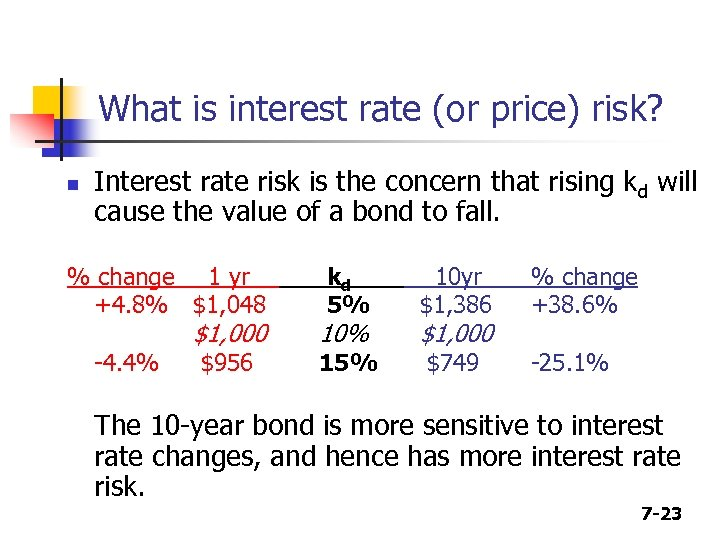What is interest rate (or price) risk? n Interest rate risk is the concern that rising kd will cause the value of a bond to fall. % change 1 yr +4. 8% \$1, 048 -4. 4% \$1, 000 \$956 kd 5% 10 yr \$1, 386 15% \$749 10% \$1, 000 % change +38. 6% -25. 1% The 10 -year bond is more sensitive to interest rate changes, and hence has more interest rate risk. 7 -23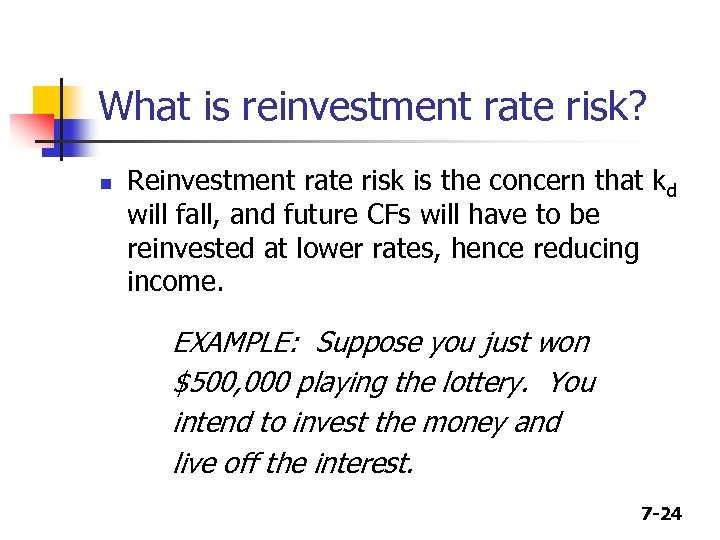What is reinvestment rate risk? n Reinvestment rate risk is the concern that kd will fall, and future CFs will have to be reinvested at lower rates, hence reducing income. EXAMPLE: Suppose you just won \$500, 000 playing the lottery. You intend to invest the money and live off the interest. 7 -24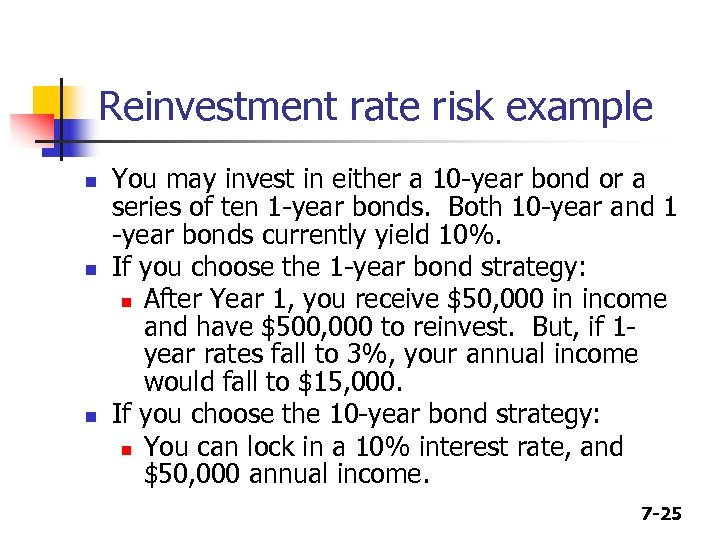Reinvestment rate risk example n n n You may invest in either a 10 -year bond or a series of ten 1 -year bonds. Both 10 -year and 1 -year bonds currently yield 10%. If you choose the 1 -year bond strategy: n After Year 1, you receive \$50, 000 in income and have \$500, 000 to reinvest. But, if 1 year rates fall to 3%, your annual income would fall to \$15, 000. If you choose the 10 -year bond strategy: n You can lock in a 10% interest rate, and \$50, 000 annual income. 7 -25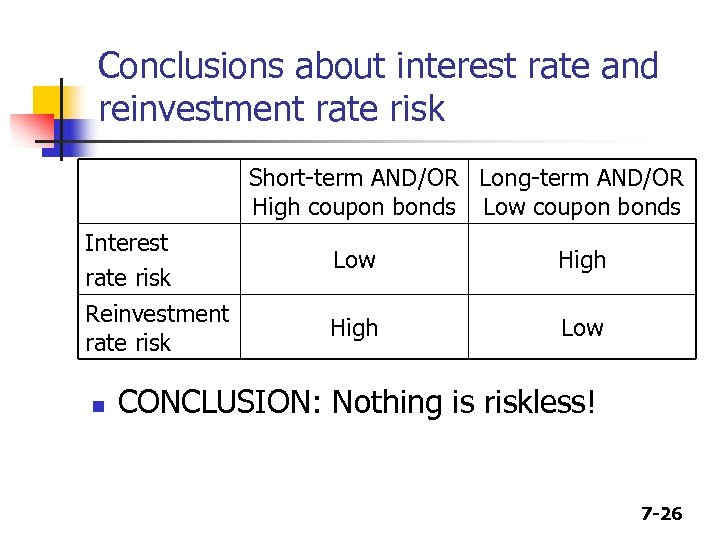Conclusions about interest rate and reinvestment rate risk Short-term AND/OR Long-term AND/OR High coupon bonds Low coupon bonds Interest rate risk Low High Reinvestment rate risk High Low n CONCLUSION: Nothing is riskless! 7 -26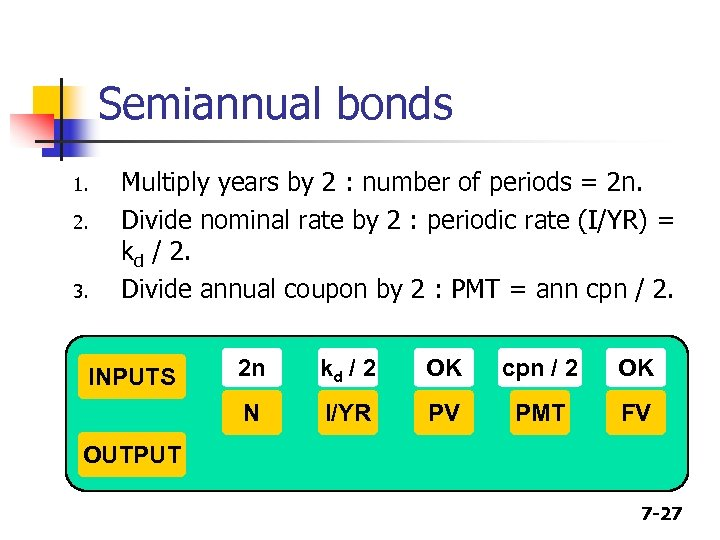Semiannual bonds 1. 2. 3. Multiply years by 2 : number of periods = 2 n. Divide nominal rate by 2 : periodic rate (I/YR) = kd / 2. Divide annual coupon by 2 : PMT = ann cpn / 2. 2 n kd / 2 OK cpn / 2 OK N INPUTS I/YR PV PMT FV OUTPUT 7 -27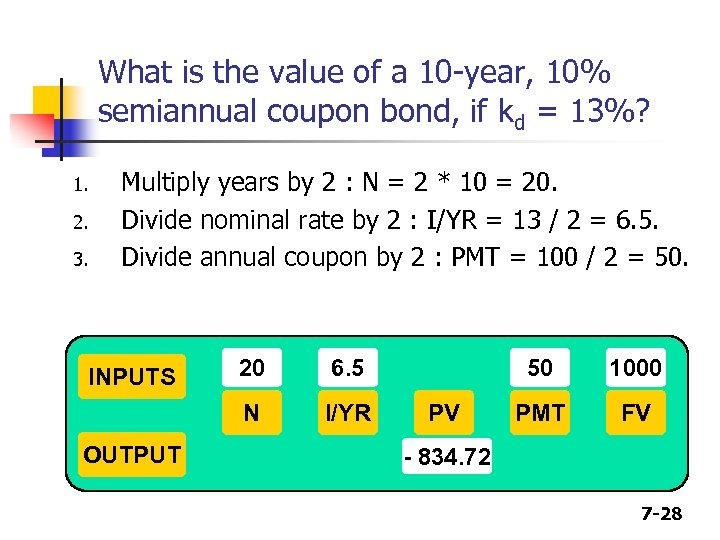What is the value of a 10 -year, 10% semiannual coupon bond, if kd = 13%? 1. 2. 3. Multiply years by 2 : N = 2 * 10 = 20. Divide nominal rate by 2 : I/YR = 13 / 2 = 6. 5. Divide annual coupon by 2 : PMT = 100 / 2 = 50. OUTPUT 20 6. 5 N INPUTS I/YR 50 PV 1000 PMT FV - 834. 72 7 -28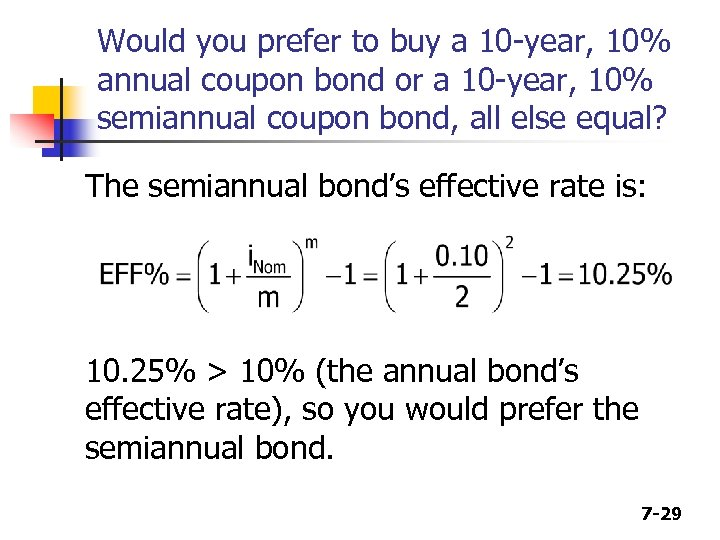Would you prefer to buy a 10 -year, 10% annual coupon bond or a 10 -year, 10% semiannual coupon bond, all else equal? The semiannual bond’s effective rate is: 10. 25% > 10% (the annual bond’s effective rate), so you would prefer the semiannual bond. 7 -29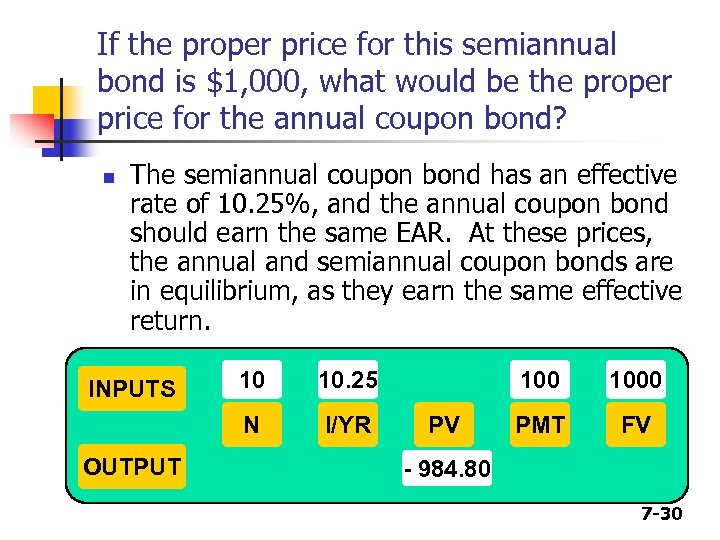If the proper price for this semiannual bond is \$1, 000, what would be the proper price for the annual coupon bond? n The semiannual coupon bond has an effective rate of 10. 25%, and the annual coupon bond should earn the same EAR. At these prices, the annual and semiannual coupon bonds are in equilibrium, as they earn the same effective return. OUTPUT 10 10. 25 N INPUTS I/YR 100 PV 1000 PMT FV - 984. 80 7 -30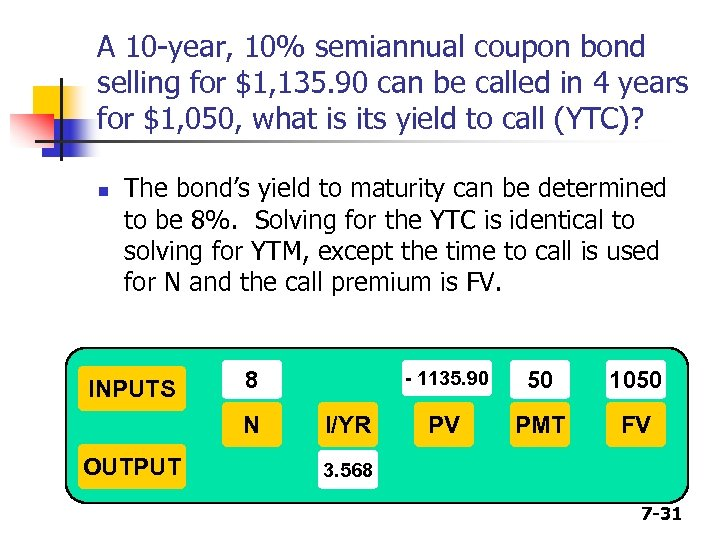A 10 -year, 10% semiannual coupon bond selling for \$1, 135. 90 can be called in 4 years for \$1, 050, what is its yield to call (YTC)? n The bond’s yield to maturity can be determined to be 8%. Solving for the YTC is identical to solving for YTM, except the time to call is used for N and the call premium is FV. INPUTS 8 N OUTPUT - 1135. 90 I/YR 50 1050 PV PMT FV 3. 568 7 -31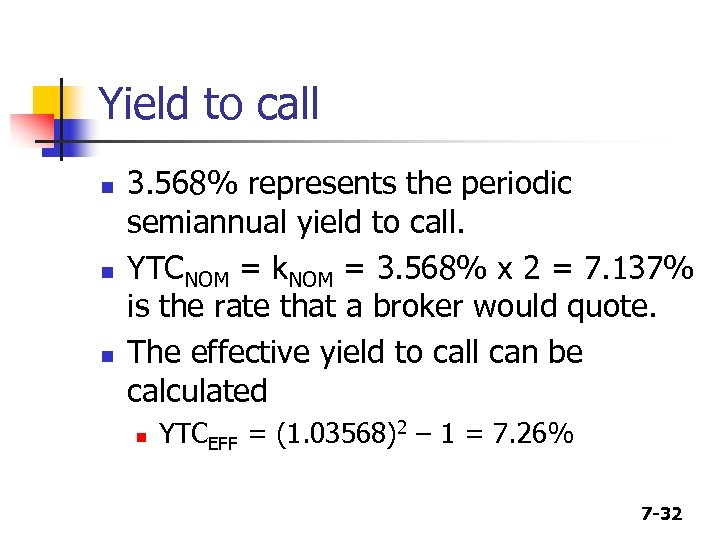Yield to call n n n 3. 568% represents the periodic semiannual yield to call. YTCNOM = k. NOM = 3. 568% x 2 = 7. 137% is the rate that a broker would quote. The effective yield to call can be calculated n YTCEFF = (1. 03568)2 – 1 = 7. 26% 7 -32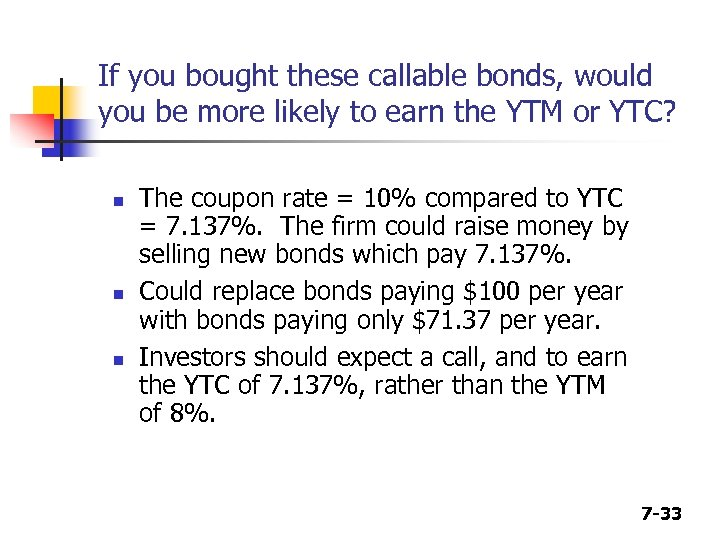If you bought these callable bonds, would you be more likely to earn the YTM or YTC? n n n The coupon rate = 10% compared to YTC = 7. 137%. The firm could raise money by selling new bonds which pay 7. 137%. Could replace bonds paying \$100 per year with bonds paying only \$71. 37 per year. Investors should expect a call, and to earn the YTC of 7. 137%, rather than the YTM of 8%. 7 -33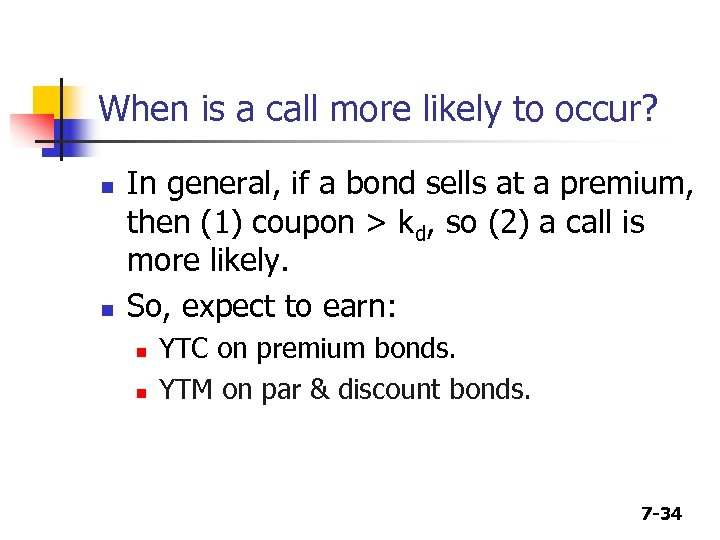When is a call more likely to occur? n n In general, if a bond sells at a premium, then (1) coupon > kd, so (2) a call is more likely. So, expect to earn: n n YTC on premium bonds. YTM on par & discount bonds. 7 -34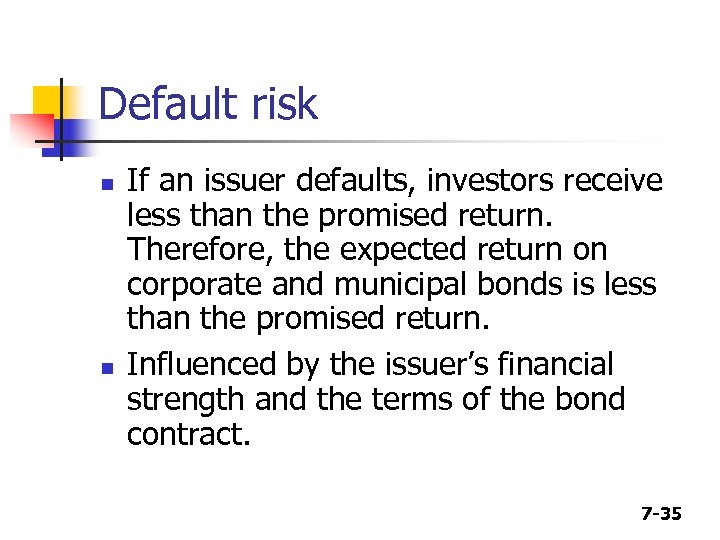Default risk n n If an issuer defaults, investors receive less than the promised return. Therefore, the expected return on corporate and municipal bonds is less than the promised return. Influenced by the issuer’s financial strength and the terms of the bond contract. 7 -35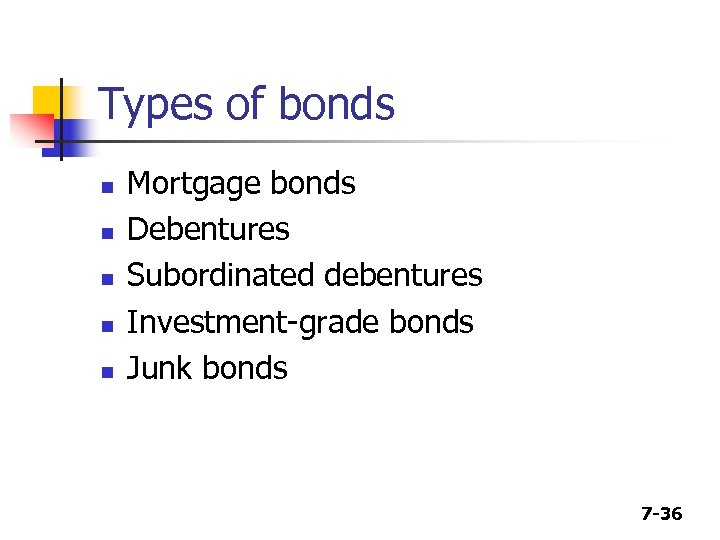Types of bonds n n n Mortgage bonds Debentures Subordinated debentures Investment-grade bonds Junk bonds 7 -36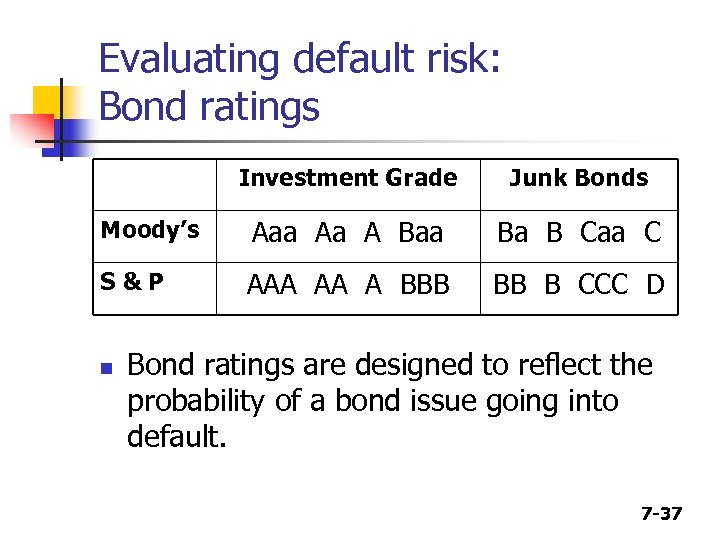Evaluating default risk: Bond ratings Investment Grade Junk Bonds Moody’s Aaa Aa A Baa Ba B Caa C S&P AAA AA A BBB BB B CCC D n Bond ratings are designed to reflect the probability of a bond issue going into default. 7 -37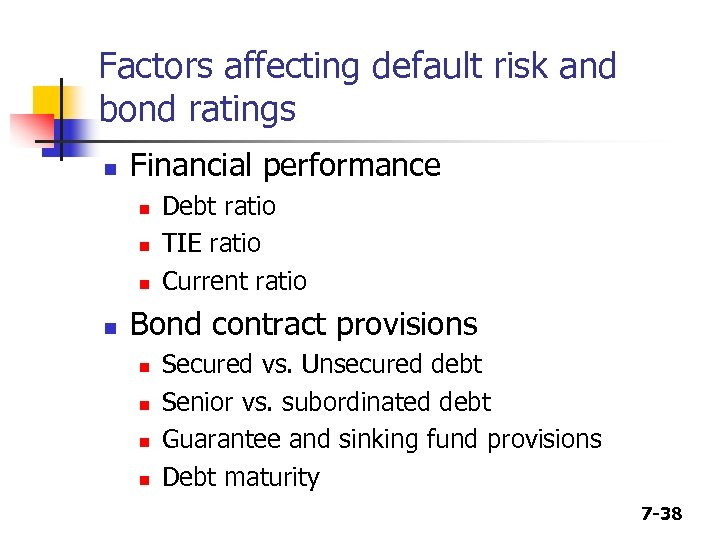Factors affecting default risk and bond ratings n Financial performance n n Debt ratio TIE ratio Current ratio Bond contract provisions n n Secured vs. Unsecured debt Senior vs. subordinated debt Guarantee and sinking fund provisions Debt maturity 7 -38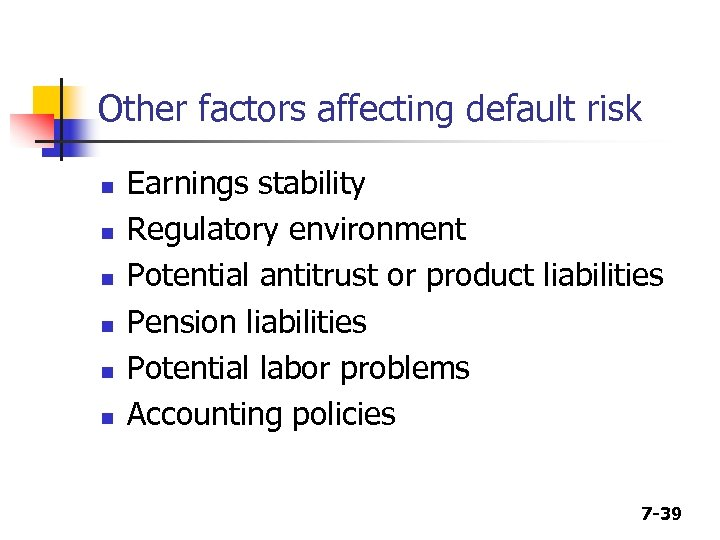Other factors affecting default risk n n n Earnings stability Regulatory environment Potential antitrust or product liabilities Pension liabilities Potential labor problems Accounting policies 7 -39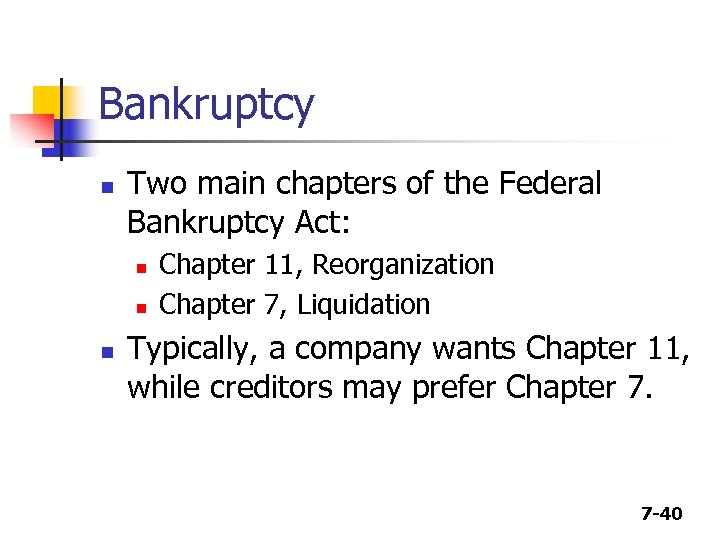Bankruptcy n Two main chapters of the Federal Bankruptcy Act: n n n Chapter 11, Reorganization Chapter 7, Liquidation Typically, a company wants Chapter 11, while creditors may prefer Chapter 7. 7 -40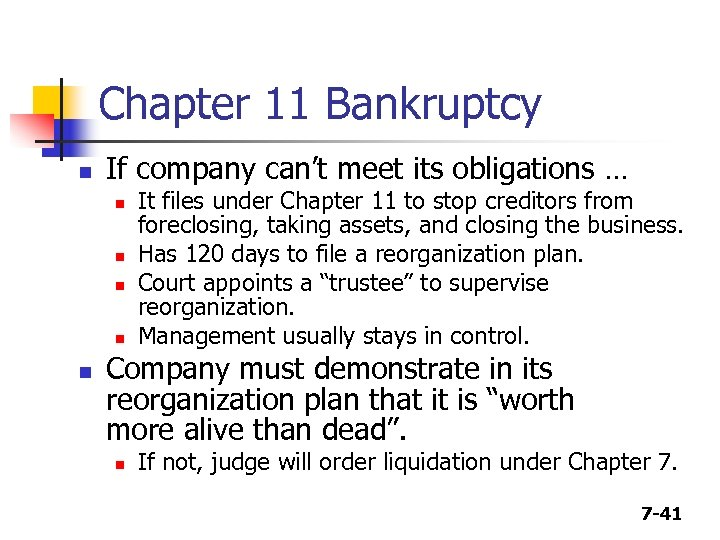Chapter 11 Bankruptcy n If company can’t meet its obligations … n n n It files under Chapter 11 to stop creditors from foreclosing, taking assets, and closing the business. Has 120 days to file a reorganization plan. Court appoints a “trustee” to supervise reorganization. Management usually stays in control. Company must demonstrate in its reorganization plan that it is “worth more alive than dead”. n If not, judge will order liquidation under Chapter 7. 7 -41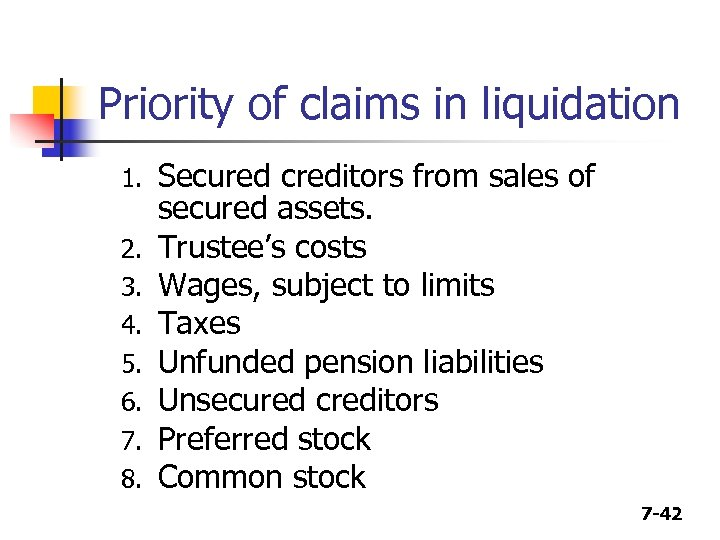Priority of claims in liquidation 1. 2. 3. 4. 5. 6. 7. 8. Secured creditors from sales of secured assets. Trustee’s costs Wages, subject to limits Taxes Unfunded pension liabilities Unsecured creditors Preferred stock Common stock 7 -42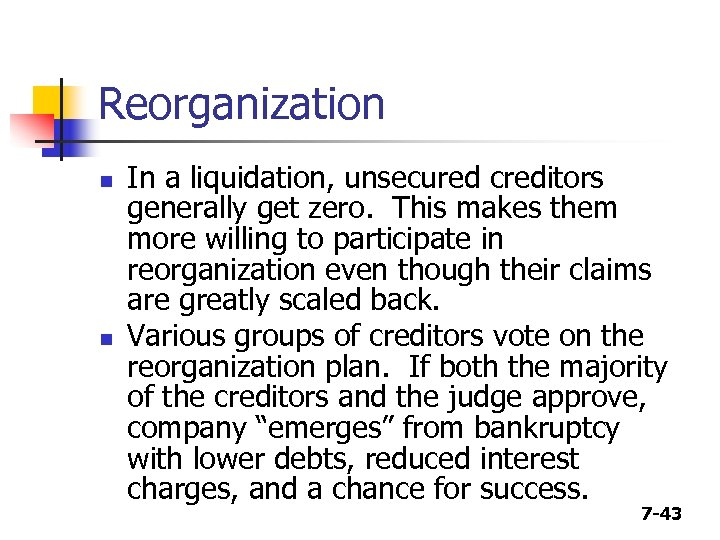Reorganization n n In a liquidation, unsecured creditors generally get zero. This makes them more willing to participate in reorganization even though their claims are greatly scaled back. Various groups of creditors vote on the reorganization plan. If both the majority of the creditors and the judge approve, company “emerges” from bankruptcy with lower debts, reduced interest charges, and a chance for success. 7 -43